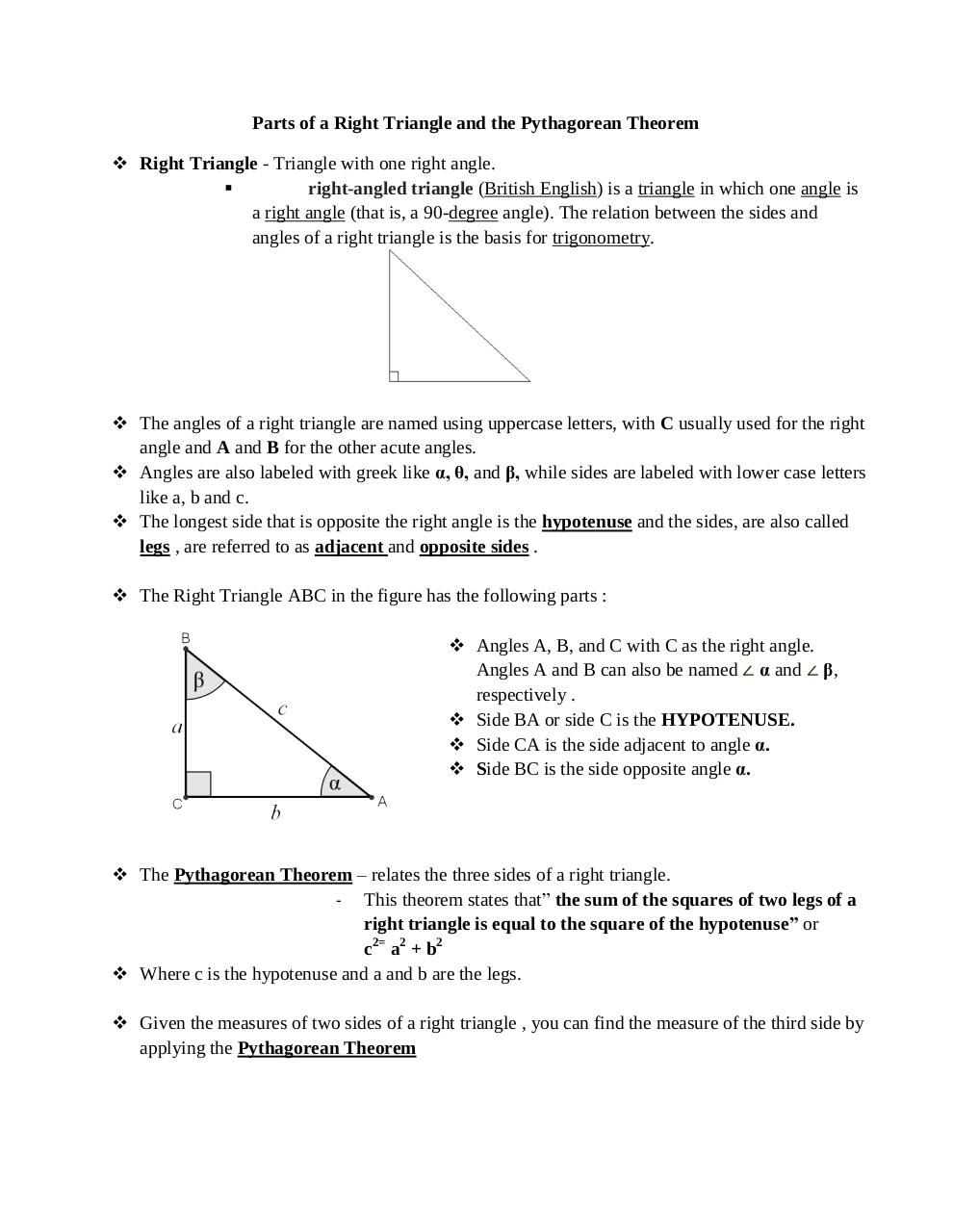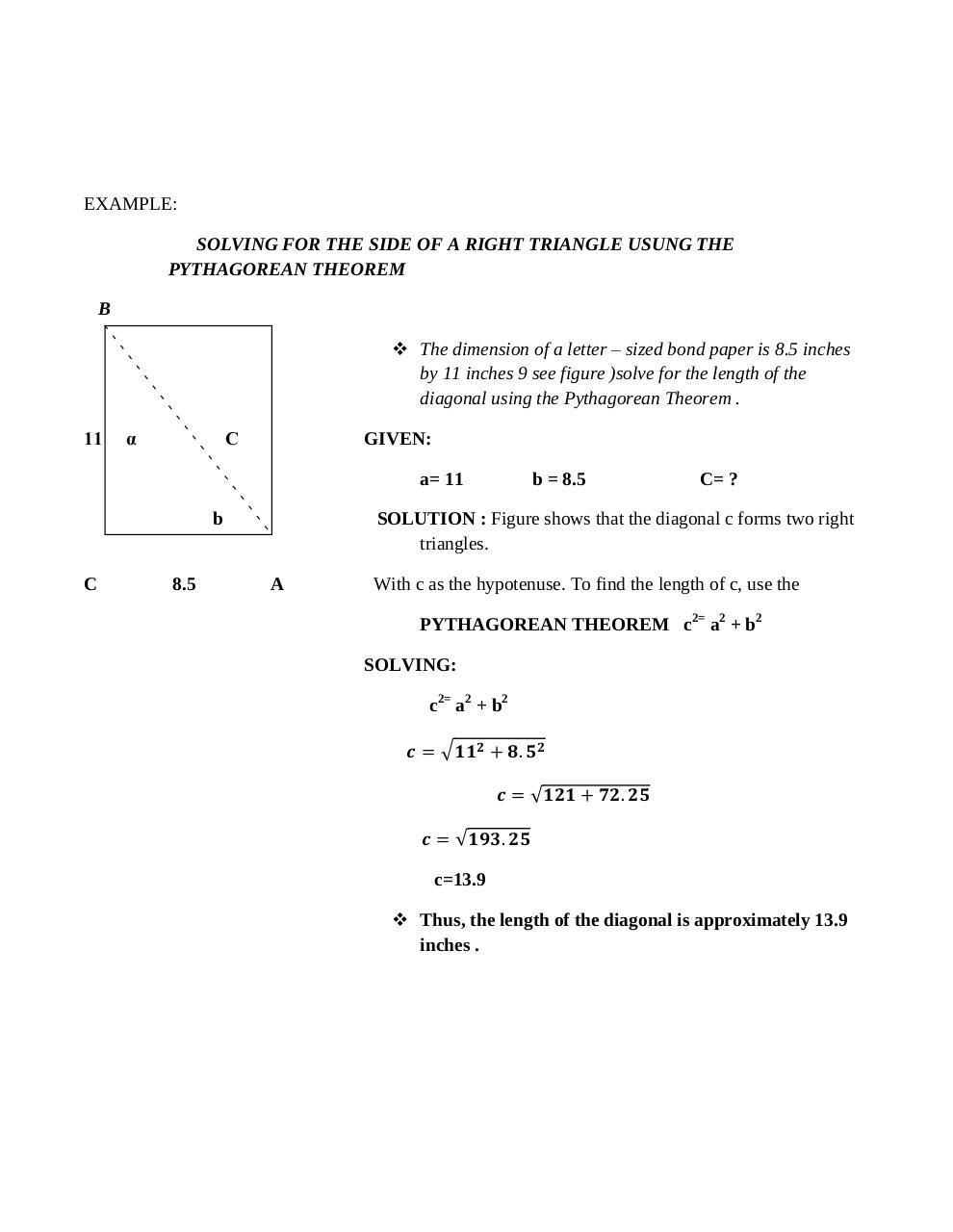# Parts of a Right Triangle and the Pythagorean Theorem .pdf

### File information

Original filename: Parts-of-a-Right-Triangle-and-the-Pythagorean-Theorem.pdf
Author: ELICO

This PDF 1.5 document has been generated by MicrosoftÂ® Word 2010, and has been sent on pdf-archive.com on 16/02/2017 at 14:45, from IP address 49.149.x.x. The current document download page has been viewed 591 times.
File size: 489 KB (2 pages).
Privacy: public file

Parts-of-a-Right-Triangle-and-the-Pythagorean-Theorem.pdf (PDF, 489 KB)

### Document preview

Parts of a Right Triangle and the Pythagorean Theorem
 Right Triangle - Triangle with one right angle.

right-angled triangle (British English) is a triangle in which one angle is
a right angle (that is, a 90-degree angle). The relation between the sides and
angles of a right triangle is the basis for trigonometry.

 The angles of a right triangle are named using uppercase letters, with C usually used for the right
angle and A and B for the other acute angles.
 Angles are also labeled with greek like α, θ, and β, while sides are labeled with lower case letters
like a, b and c.
 The longest side that is opposite the right angle is the hypotenuse and the sides, are also called
legs , are referred to as adjacent and opposite sides .
 The Right Triangle ABC in the figure has the following parts :
 Angles A, B, and C with C as the right angle.
Angles A and B can also be named ∠ α and ∠ β,
respectively .
 Side BA or side C is the HYPOTENUSE.
 Side CA is the side adjacent to angle α.
 Side BC is the side opposite angle α.

 The Pythagorean Theorem – relates the three sides of a right triangle.
- This theorem states that” the sum of the squares of two legs of a
right triangle is equal to the square of the hypotenuse” or
c2= a2 + b2
 Where c is the hypotenuse and a and b are the legs.
 Given the measures of two sides of a right triangle , you can find the measure of the third side by
applying the Pythagorean Theorem

EXAMPLE:
SOLVING FOR THE SIDE OF A RIGHT TRIANGLE USUNG THE
PYTHAGOREAN THEOREM
B
 The dimension of a letter – sized bond paper is 8.5 inches
by 11 inches 9 see figure )solve for the length of the
diagonal using the Pythagorean Theorem .
11

α

C

GIVEN:
a= 11

b

C

8.5

b = 8.5

C= ?

SOLUTION : Figure shows that the diagonal c forms two right
triangles.
A

With c as the hypotenuse. To find the length of c, use the
PYTHAGOREAN THEOREM c2= a2 + b2
SOLVING:
c2= a2 + b2

c=13.9
 Thus, the length of the diagonal is approximately 13.9
inches .#### HTML Code

Copy the following HTML code to share your document on a Website or Blog

#### QR Code### Related keywords